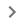TO_PERCENT

Converts a provided number to a percentage.

Sample Usage

TO_PERCENT(A2)

TO_PERCENT(0.40826)

Syntax

TO_PERCENT(value)

• value - The argument or reference to a cell to be converted to a percentage.

• If value is a number or a reference to a cell containing a numeric value, TO_PERCENT returns value converted to a percentage, with the standard interpretation that 1 = 100%.

• If value is not a number or a reference to a cell containing a numeric value, TO_PERCENT returns value without modification.

Notes

• Because dates and percentages are both numbers, TO_PERCENT will convert dates to percentages successfully. However, these conversions are not typically meaningful.

• TO_PERCENT is equivalent to clicking FormatNumberPercent from the menu bar.

UNARY_PERCENT: Returns a value interpreted as a percentage; that is, `UNARY_PERCENT(100)` equals `1`.

TO_TEXT: Converts a provided numeric value to a text value.

TO_PURE_NUMBER: Converts a provided date/time, percentage, currency or other formatted numeric value to a pure number without formatting.

TO_DOLLARS: Converts a provided number to a dollar value.

TO_DATE: Converts a provided number to a date.

N: Returns the argument provided as a number.